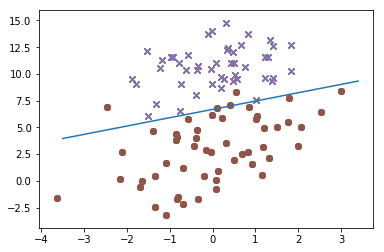Logistic 回归就是来预测二分类 0, 1 的一种回归学习算法。Logistic 回归与一般的线性回归主要不同是选择假设函数不一样。

#### 算法实现

'''

'''
import numpy as np
import matplotlib.pyplot as plt

# 加载数据
fr = open(file)
dataset = []
labels = []
lineNums = line.strip().split()
labels.append(int(lineNums[-1]))
dataset.append([1.0, float(lineNums), float(lineNums)])
return dataset, labels

# 计算 Sigmoid hypothesis value
def calcHypothesis(z):
return 1 / (1 + np.exp(-z))

# 批量梯度上升算法
def batchGradientAscend(dataset, labels, maxIter = 500, alpha = 0.001):
dataset = np.mat(dataset)
labels = np.mat(labels).transpose()
m, n = dataset.shape
weights = np.ones((n, 1))
for i in range(maxIter):
error = labels - calcHypothesis(dataset * weights)
weights = weights + alpha * dataset.T * error
return weights

def plotLinear(dataset, labels, weights):
dataset = np.mat(dataset)
labels = np.array(labels)
negLabelsIndices = labels == 0
posLabelsIndices = labels == 1
negLabels = dataset[negLabelsIndices]
posLabels = dataset[posLabelsIndices]
plt.scatter(negLabels[:, 1], negLabels[:, 2], marker='x')
plt.scatter(posLabels[:, 1], posLabels[:, 2], marker='o')
x = np.arange(-3.5, 3.5, 0.1)
y = (-weights - weights * x) / weights
plt.plot(x, np.squeeze(np.asarray(y)))
plt.show()


dataset, labels = loadDataset('./data/testSet.txt')
plotLinear(dataset, labels, weights)



#### 运行结果labels = [1, 0, 1, 0, 1]
amat = np.mat(labels)
arr = np.array(labels)
print(amat, amat.shape)
print(arr, arr.shape)

[[1 0 1 0 1]] (1, 5)
[1 0 1 0 1] (5,)